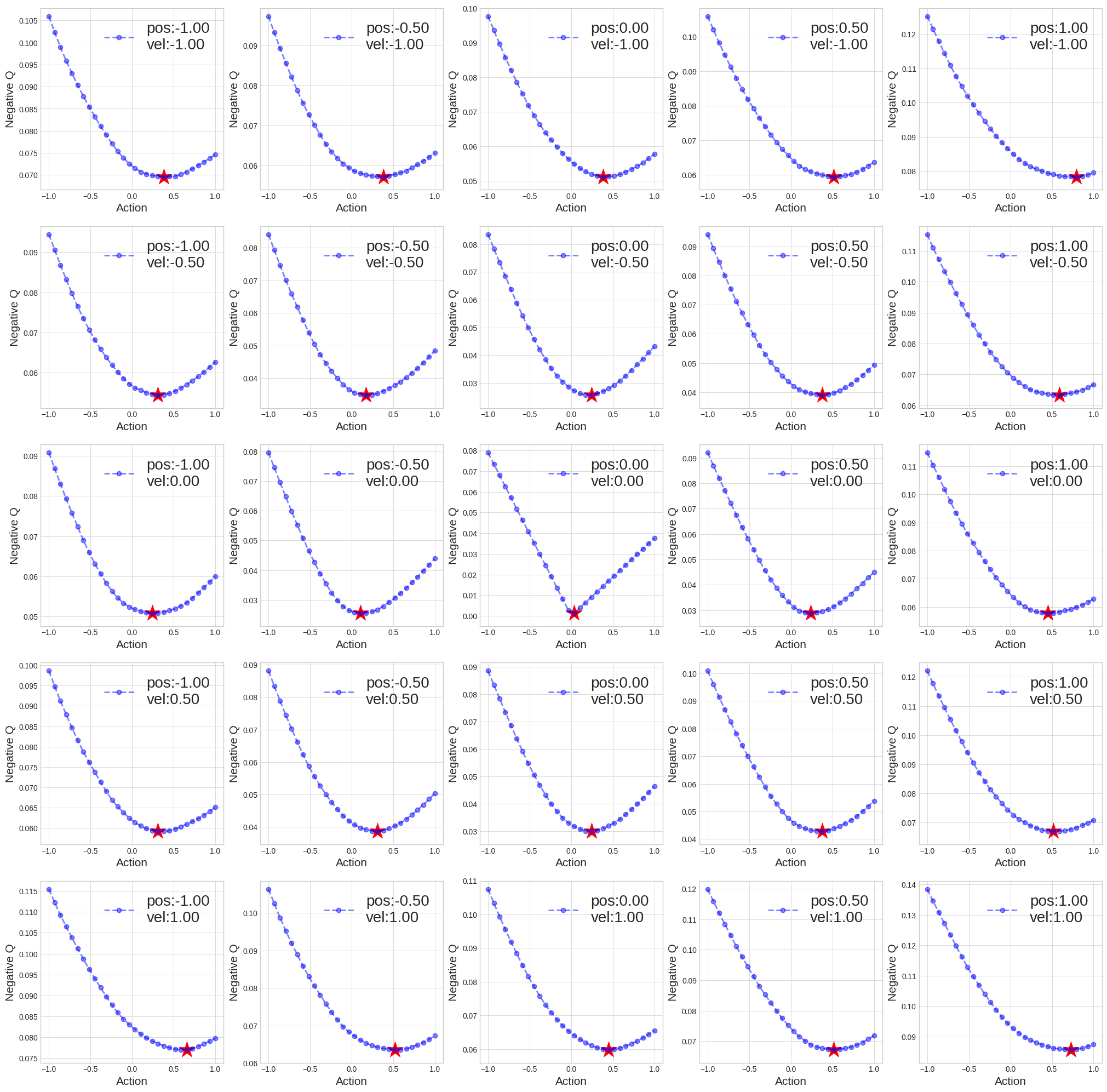# Continuous-Action Reinforcement Learning With Input Convex Neural Networks

Last updated: May 23, 2018

Input Convex Neural Network (ICNN) is a neural network that has convexity in a subset of its input. In this post, I give a brief review of the model in the context of continuous action reinforcemen learning.

### Motivation

Many inference problems are non-convex, especially when their objective functions are represented by deep neural networks. They are generally hard to solve efficiently because of potentially exponential number of stationary points with parsimonious saddle points. A local optimization method such as Gradient Descent can only guarantee the convergence to a stationary point, not necessarily the true global solution.

To better motivate the introduction of ICNN, let us suppose a given task requires solving the following optimization problems for a sufficiently large number of times with varying input values of x and non-convex $f$.

If $y$ is discrete, solving for $y^\star$ is $O(|Y|)$ where an exhaustive search is computationally feasible. If $y \in |Y|^d$ with a large $d$, or $y$ is continuous, we are quite out of luck with an exhaustive search. One may also consider discretizing continuous-valued $y$ but it requires good heuristics that are problem dependent.

### What is ICNN

Below, we define ICNN convex fully in input and partially in input.

#### Fully Input Convex Neural Networks (FICNN)

FICNN is a feedforward neural network with additional constraints as below.

where $\phi_i$ represents activation functions in layer i, $z_i$ represents the $i^{th}$ activated layer, $y$ constitutes the input and $f$ the function approximation we wish to learn, $\phi’$ represents its derivative with respect to the function’s inputs. Finally, $\mathbb{C}$ is the set of all convex functions and $W_i^{(y)}y_i$ is the term for passthrough layer.

A sufficient condition for the network to be convex in $(\mathbf{y})$) is that $\phi$ is a convex and non-decreasing function and that all $W^{(z)}_{1:K}$ are non-negative. Though this certainly restricts the expressiveness of the network and the choice of activation functions, in practice, we claim this does not come up as an insurmountable challenge. With weights forced to being non-negative, the network loes its ability to learn an identity mapping between layers, thus affecting the non-linear expressive power of the standard neural networks.

To remedy the restricted representation power, the paper proposed adding pass-through layers that directly connect the inputs $y$ to each hidden layer to learn an identity mapping. It is also worth noting that there are no constraints on the weights of the pass-through layers. Regarding the choice of activation functions, popular activation functions such as Rectified Linear Units(ReLU) or Scaled Exponential Linear Units (SeLU) and max pooling all fit into our criteria.

#### Partially Input Convex Neural Networks (PICNN)

Unlike FICNN that is convex over both $x, y$, we consider a generalization of FICNN that has convexity in a subset of the inputs, $y$. Hence, Partial Input Convex Neural Network (PICNN).

where $u_i$, $z_i$ denote the hidden layers for the non-convex and convex channels respectively, $\odot$ denotes the Hadamard product and the function. PICNN in principle has larger expressiveness than FICNN.

### Continuous Control with Deep Reinforcement LearningA training result of PICCN for OpenAI MountainCar problem. Source

Continuous control reinforcement learning problems require solving the following inference problems repeatedly and sequentially:

where $s$ denotes state, $a$ action and $Q(s,a)$ action value function. Notice the negative sign applied to keep the problem in a consistent formulation as in the introduction. The inference is almost intractable as it is. There are two state-of-the-art methods generally considered for the problem: Deep Deterministic Policy Gradient (DDPG) and Normalized Advantage Functions (NAF).

DDPG directly parametizes a deterministic policy $\pi_\theta: \mathcal{S} \mapsto \mathcal{A}$ with its corresponding performance function, defined as expected culumulative return given a policy: $J(\theta) = \mathbb{E}_{\pi}[\sum_{t=1}^\infty r(s_t,a_t)]$. With a deterministic policy, we can replace $a^* = \arg\min_{a} -Q(s,a)$ with $a^\star = \pi_\theta(s)$. The paper showed the Deterministic Policy Gradient converges to the stochastic policy gradient. And the deterministic Policy Gradient can be estimated even more easily and since we are choosing actions using a policy, one can sidestep the $\arg\min Q$ bottleneck.

NAF decomposes the Q-values into value function of the state and an advantage function such that $Q(s,a) = V(s) + A(s,a)$. NAF constraints $A(s,a)$ such that it is concave quadratic with respect to action and hence always gives a closed form solution for $\arg\min_{a} -Q(s,a)$. Notice $V(s)$ does not depend on $a$ and therefore can be ignored. ICNN shares the central idea of making $Q$ or its components convex with respect to action.

### When Is ICNN Useful?

The way ICNN attempts to tackle the difficult inference task above is simple: it aims to formulate the problem as an approximate yet convex problem, hoping the solution to the approximate formulation is acceptably good. Though not explicitly mentioned in the original paper of ICNN, I think ICNN is applicable when one of the following two conditions hold. The first is when a given problem has some hidden convexity intrinsic to it–hidden in the sense the problem is convex with respect to some proper subset of the input which is hard to know a priori. The seond is when multi-modality is not really a problem–a strong global optimum (the mode) exists.